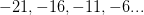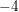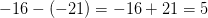# SSAT Upper Level Math : Sequences and Series

## Example Questions

### Example Question #11 : Sequences And Series

What is the next number in the sequence?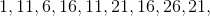_______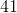Explanation:

In order to find the next number in the sequence, take a look at the patterns and common differences between the existing numbers in the sequence. Starting with, we addto getand then subtractto get.

By the time we get to, we have subtractedfromto complete the cycle of common differences. We will therefore addtonext, getting the missing number.

### Example Question #12 : Sequences And Series

What is the next number in the sequence?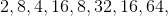_______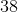Explanation:

In order to find the next number in the sequence, take a look at the patterns and common differences between the existing numbers in the sequence. Starting at the beginning, we multiplybyto getand then divide byto getWe multiply the secondin the sequence byto get, so by the logic of the sequence we will be dividing byto get the missing number.

### Example Question #1 : Common Difference In Sequences

Find the common difference for the arithmetic sequence: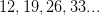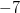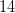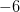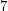Explanation:

Subtract the first term from the second term to find the common difference.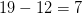### Example Question #1571 : Ssat Upper Level Quantitative (Math)

Find the common difference for the arithmetic sequence: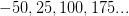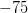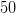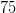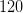Explanation:

Subtract the first term from the second term to find the common difference.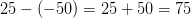### Example Question #11 : Sequences And Series

Find the common difference for the arithmetic sequence: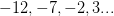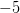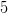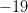Explanation:

Subtract the first term from the second term to find the common difference.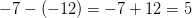### Example Question #1 : How To Find The Common Difference In Sequences

Find the common difference for the arithmetic sequence: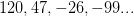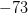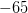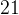Explanation:

Subtract the first term from the second term to find the common difference.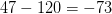### Example Question #1 : How To Find The Common Difference In Sequences

Find the common difference for the arithmetic sequence: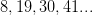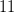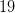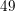Explanation:

Subtract the first term from the second term to find the common difference.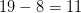### Example Question #11 : Common Difference In Sequences

Find the common difference for the arithmetic sequence: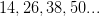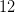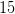Explanation:

Subtract the first term from the second term to find the common difference.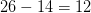### Example Question #11 : Common Difference In Sequences

Find the common difference for the arithmetic sequence: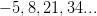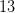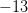Explanation:

Subtract the first term from the second term to find the common difference.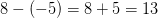### Example Question #12 : Common Difference In Sequences

Find the common difference for the arithmetic sequence: